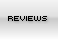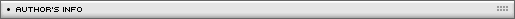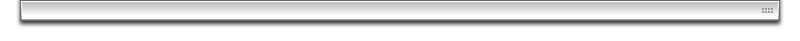Isometric 3D math
[ July 22, 2004 ] by Eric Lin
Learn the math behind an isometric 3d engine

Last year, Mike in Xpress sent me an fla. We needed to correct this swapdepths thing.

From the movie above, we notice that, the player (represented by yelow block) does not show correct depths when he goes midway behind the west two green blocks.
When the player moves, we need to swap the depths of the player correctly when he is hidden by a block or in front of the block. The most popular depths algorithm is y (row) dominant policy. The depth value of the tile depends on what row and col (y or x) it is at.

tile_depth=((col_count)*y+x)*10;

And the depth value of the player is just 1 higher than the tile he stands on.
However, this algorithm is completely y dependent. When the player stands midway and covering 2 different y value, it is difficult to handle the depths.
At anytime, when the player stands at a tile (x,y), the depths of the player must be a value higher than (x-1,y); Now the player is half way, he should be hidden by blocks both at (x+1,y) and (x+1,y-1); So, we got to make the depths less than (x+1,y-1) and greater than (x-1,y); Now we try to replace the col_count by K;

```K*(y-1)+(x+1)   >    K*y+(x-1)

K*y-K+x+1   >    K*y+x-1

-K+1   >   -1

2   >    K;
```

If we use the equation to assign the depths of tiles, to make the depths correct, K should be smaller than 2. For our equation, if K represent col_count, it is impossible to achieve the correct depths swapping since our col_count is not less than 2;

You might wonder, if we need K<2 why not use 1/row_count, so the equation will be depth_value=(y+row_count*x)*10 ?

OK, this will make it correct when player stands midway between 2 y value. But, again, this will cause trouble when the player stands covering two different x values.

So, we also know that, to make depths sorting correct, 1/K should also be less than 2; In other word, if we set K=a/b; then a<2*b and also b<2*a;

Now, we are going to change this equation. We need an equation so that a<2*b and also b<2*a ; How about setting K=101/100 or 100/101; Our new equation will be

depth_value=(100*y+101*x)*10;

That is the way I swap depths in my isometric game.

Wait ! Why do I pick such weird number ? Can we use 3/2 ? so the equation will be depth_value=(3*x+2*y)*10 ?

Theoretically, yes, that would work. However, we have many many tiles and it is essential that each tile should have a "unique" depth value. For an equation as 3*x+2*y , we will get a conflict that, the tile at (6,6) (4,9) ,(8,3) will be swapped to the same depths. Attach a movie to the same depth level will delete the previous one. If we attach them to unique depths and later rearrange the depths by this equation, when we make this tile swapDepths(30), then we will pass the old depths value to the tile that occupies at depths 30. No deleting occurrs but it messes up depths value of tiles.

For "unique" depths value, we need to clear the math here: What x, y will give the same depths ?

Let's see the equation: ax+by=a(x+dx)+b(y+dy), here dx and dy are integers;

We get dx= -(b/a)*dy; or dy= -(a/b)*dx;

So, if we remove the common factorial of a and b, we will see that: When dx can be divide by a or dy can be divided by b, then we will find conflict. To make it simply, for equation depth=ax+by, The tile depth at (x,y) will get depths conflict with next a col or next b row;

For example, an equation of depth= 3*x+2*y;

a is 3, b is 2; When dy=-2,0,2,4,6,8......, conflict occurrs..; Taking dy=-2; dx=-(3/2)*(-2), that is 3;

(3,3) will conflict with next 3 row, that is (3-2,3+3) ==> (1,6);

(4,3) will conflict with next 3 row, that is (4-2,3+3) ==> (2,6);

(5,3) will conflict with next 3 row, that is (5-2,3+3); ==> (3,6); etc;

if we take dy=-4, dx=-(3/2)*(-4); that is 6; So, (5,3) will conflict with also (5-4,3+6), that is (1,9) that is next 6 row, the row of next 3 of next 3;

How to avoid conflict ?

Well, if we make a=100;b=101; To get conflict, the dx needs to be divided by 101. or the dy needs to be divided by 100; Make it simply, the conflict will occurr for next 101 col or next 100 row. It will be safe if our map is only 30x30 tiles. In other word, if our map is 30x30, we can make depths=(31*x+32*y)*10; That is safe enough;

Could we use a=150,b=120 ? No, for the equation, we need to remove the common factorial, 150/120 is the same as 5/4; Conflicts will occurr after 5 rows or 4 cols.

That is all the math about the swap depths.

The last thing: Why we multiply them by 10 in this equation ? That equation is for tile. We need depth room for player or standing tile. Multiplying by 4 will usually enough, because we can set the depth of player as d-1 and standTile as d-2;Name: Eric Lin Location: Taiwan Age: 46 Flash experience: Since Flash 5, about 4 years Job: neurosurgeon (a doctor who operates people's brain) Website: http://ericlin2.tripod.com/| Homepage | News | Games | Articles | Multiplayer Central | Reviews | Spotlight | Forums | Info | Links | Contact us | Advertise | Credits || www.smartfoxserver.com | www.gotoandplay.biz | www.openspace-engine.com | gotoAndPlay() v 3.0.0 -- (c)2003-2008 gotoAndPlay() Team -- P.IVA 03121770048